# Selina Solutions Concise Maths Class 7 Chapter 1: Integers

Selina Solutions Concise Maths Class 7 Chapter 1 Integers help students understand that the integers are a set of both natural numbers and negatives of them including zero. The various operations on the integers are the main topics, which are discussed in brief under this chapter. In order to know a better way of solving problems easily, students can use the solutions PDF. These solutions are designed by an expert set of faculty at BYJU’S, keeping in mind the understanding capacity of students. For better conceptual knowledge, students can make use of Selina Solutions Concise Maths Class 7 Chapter 1 Integers PDF, from the link which is given below.

This chapter provides an introduction to integers, multiplication and division of integers, removal of brackets. The problems will mainly help students develop a better idea about the fundamental concept of integers, which are important for the exams. Students who aspire to perform well in the annual exam are advised to solve the exercise wise problems daily.

## Selina Solutions Concise Maths Class 7 Chapter 1: Integers Download PDF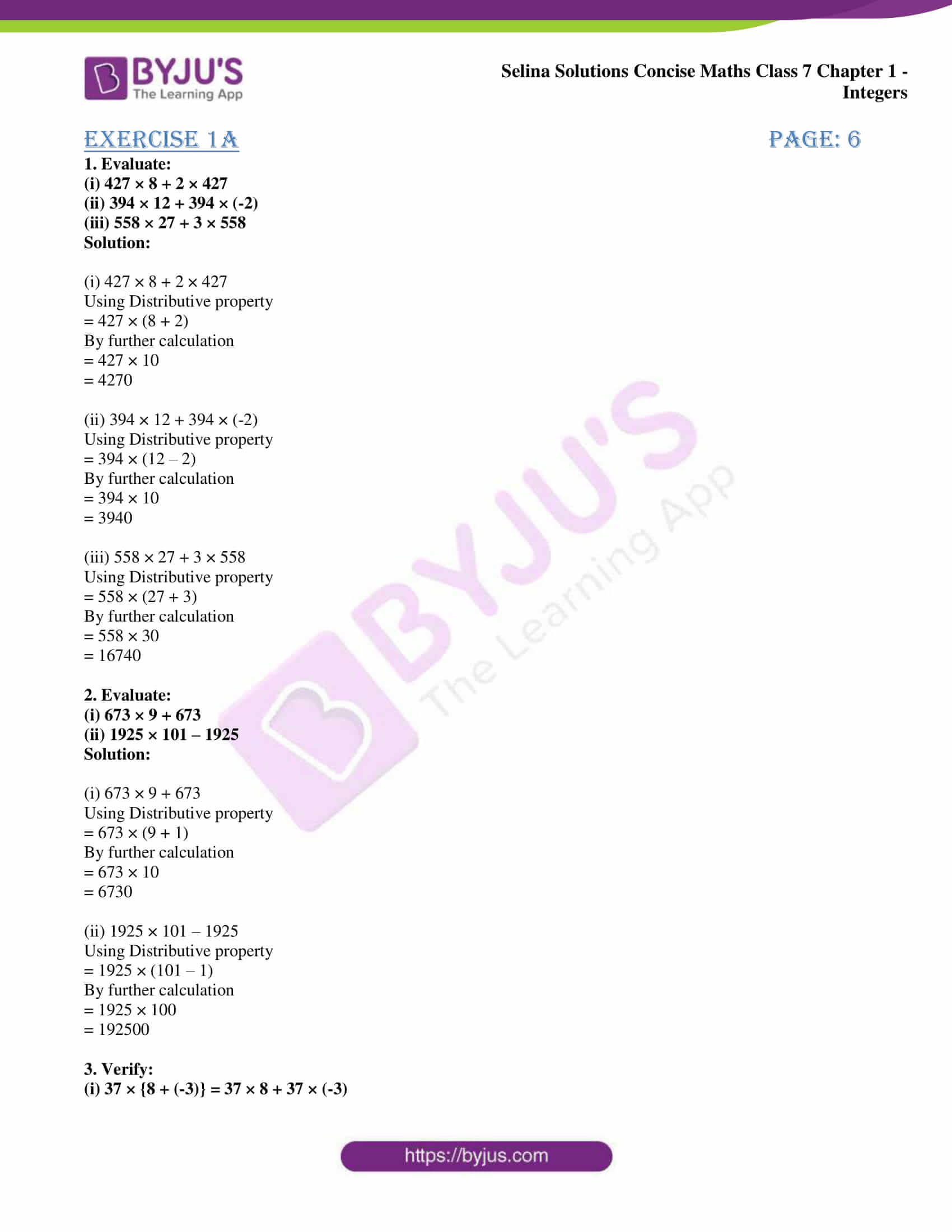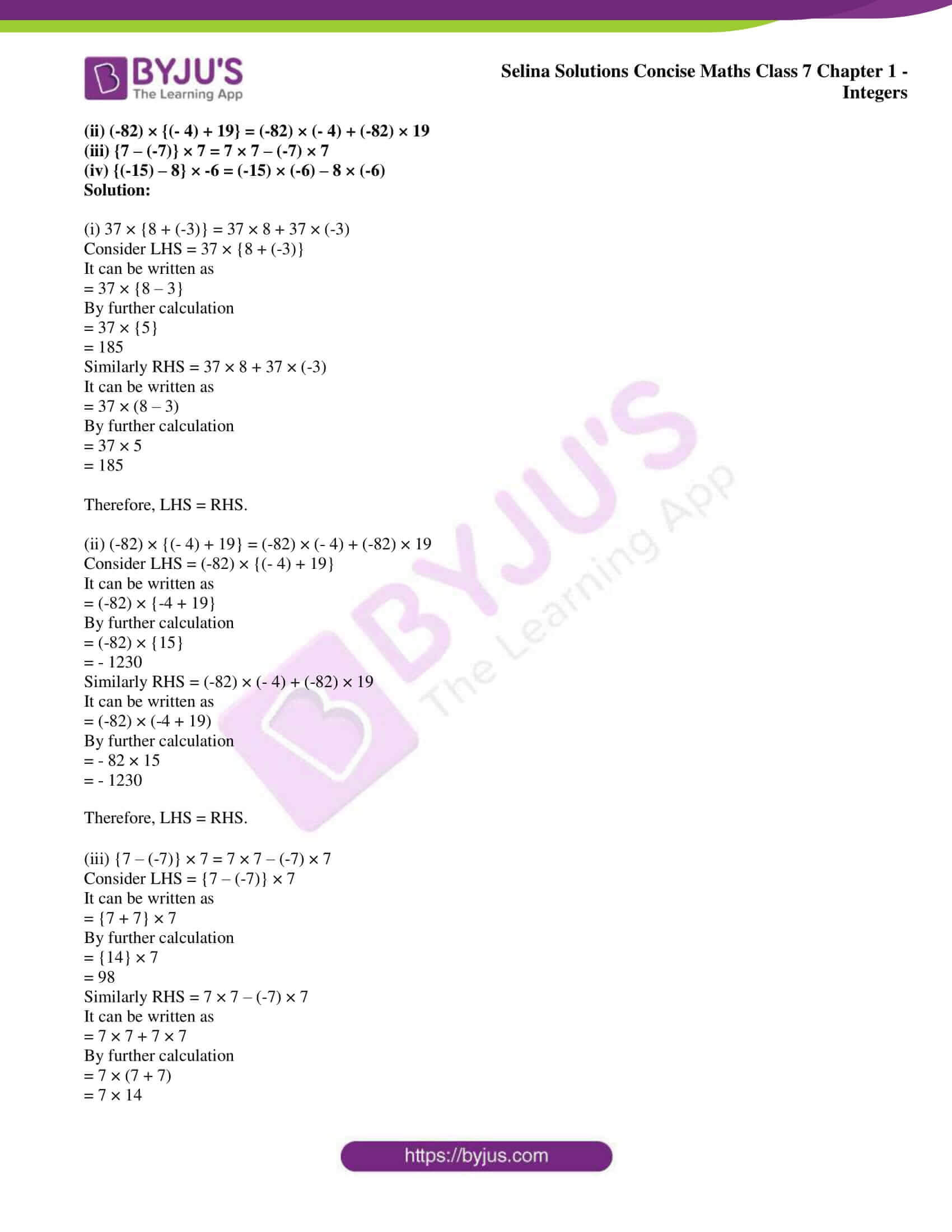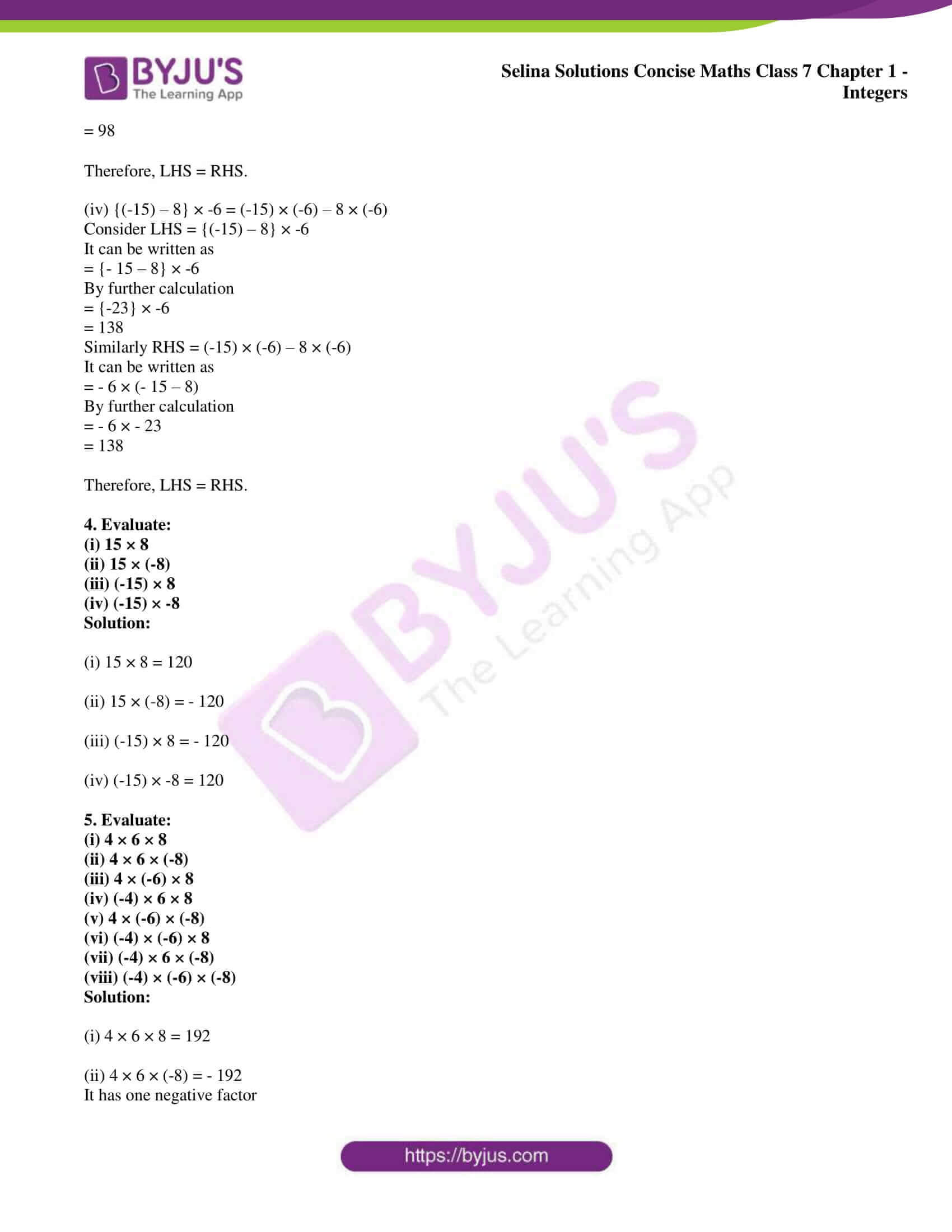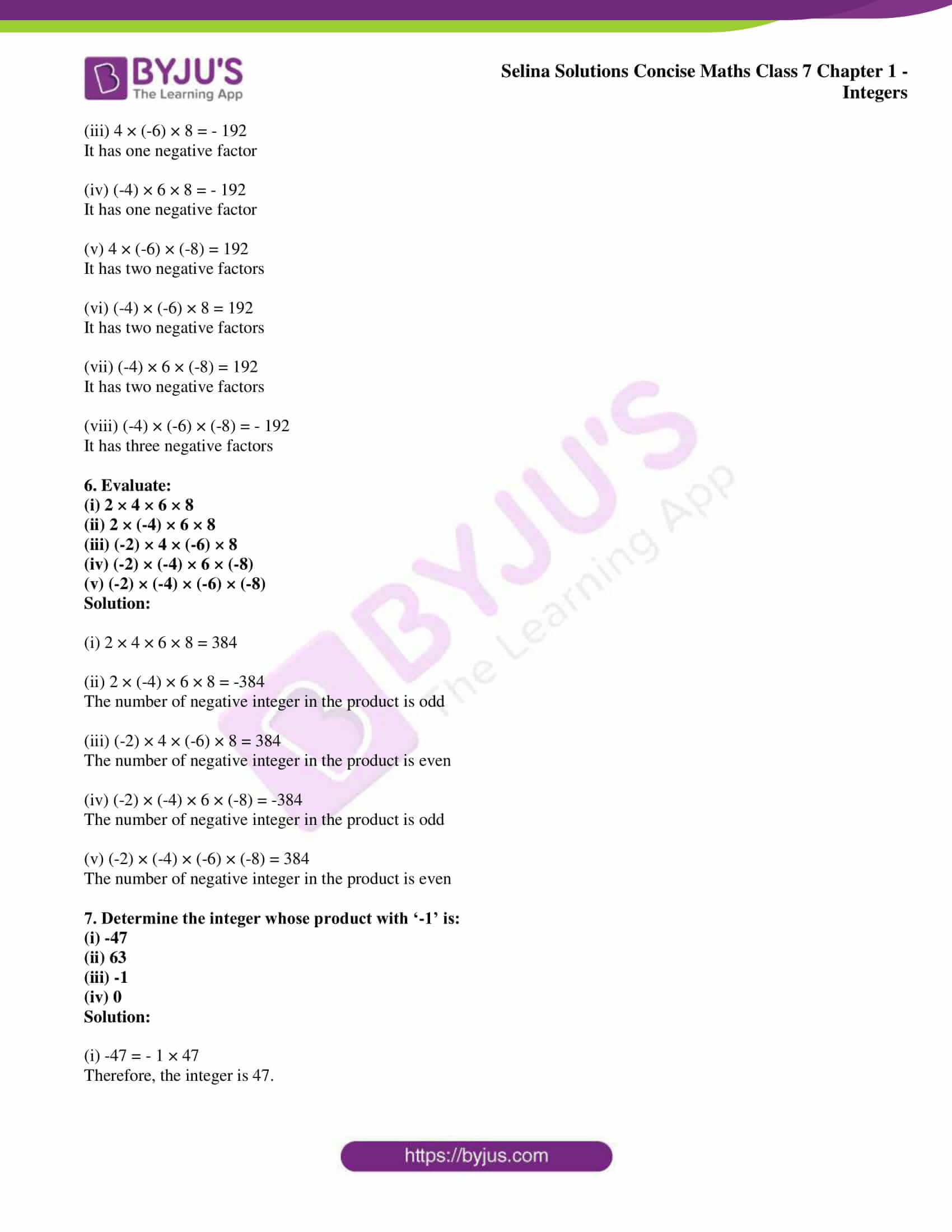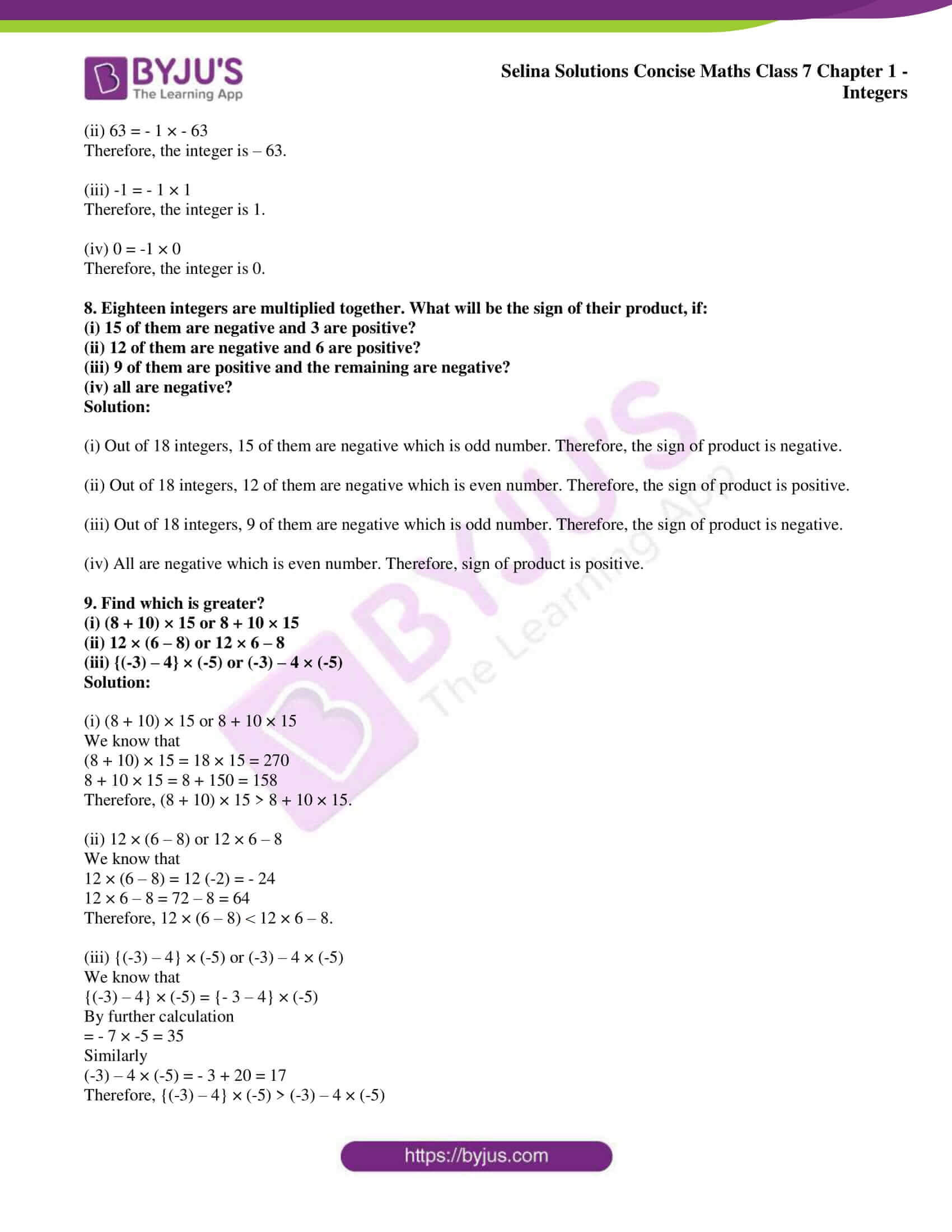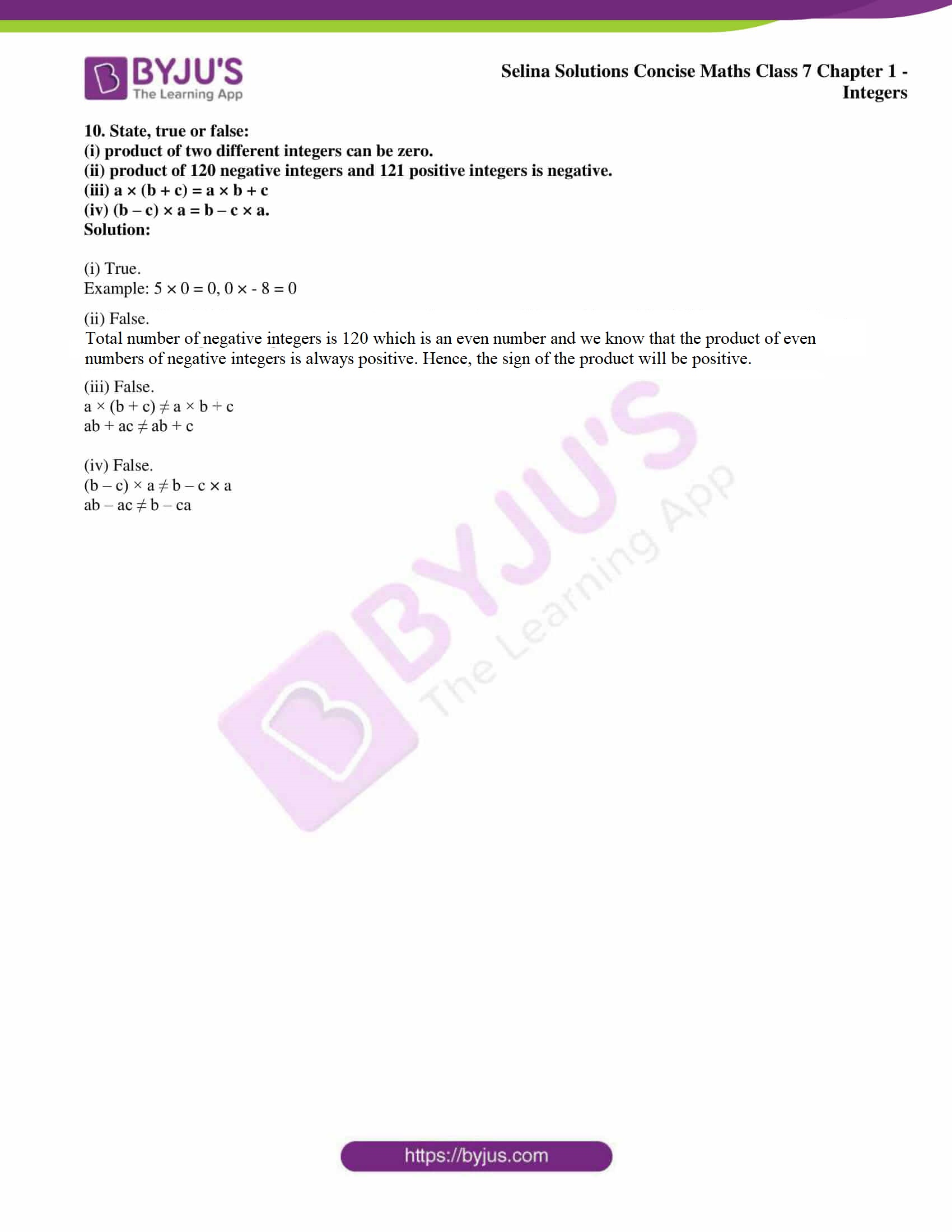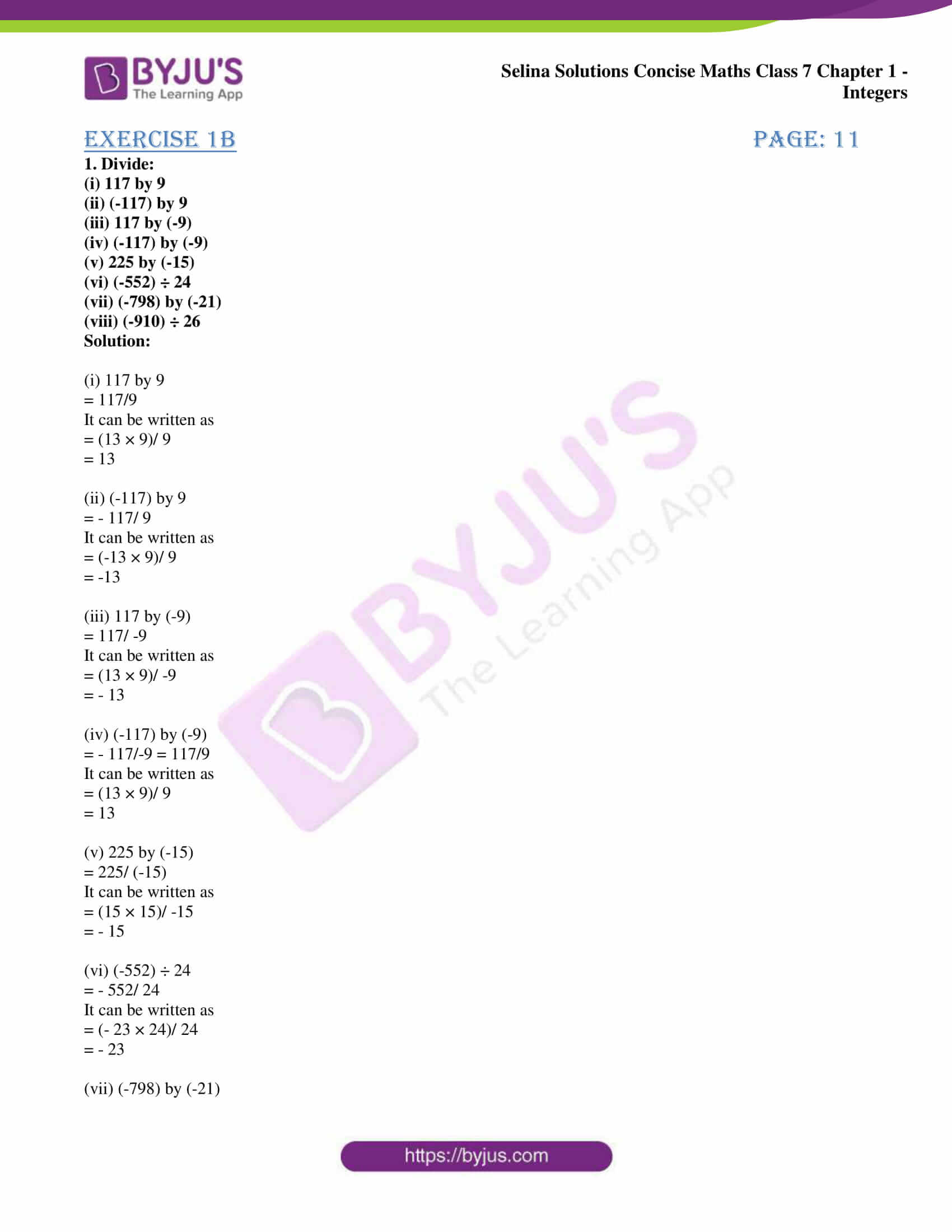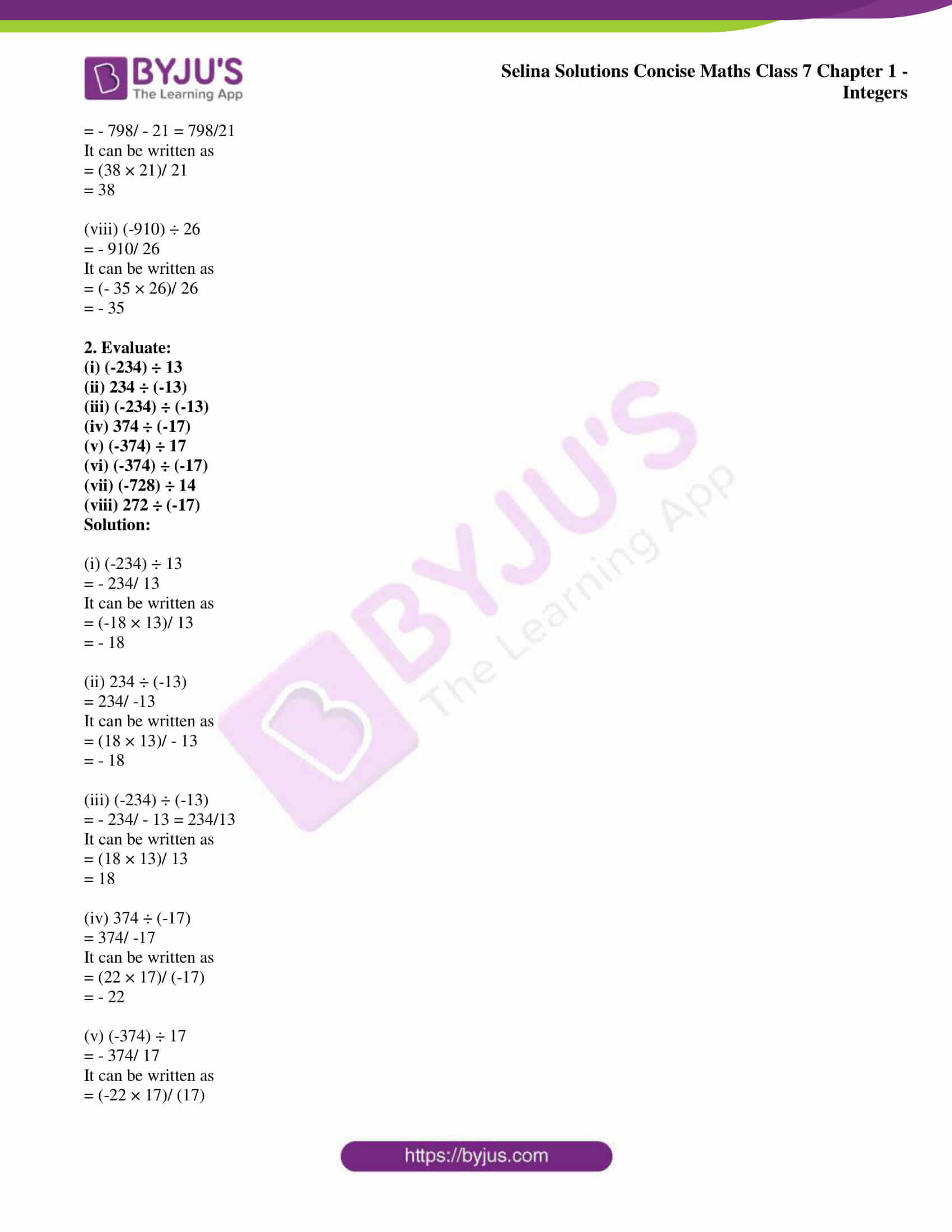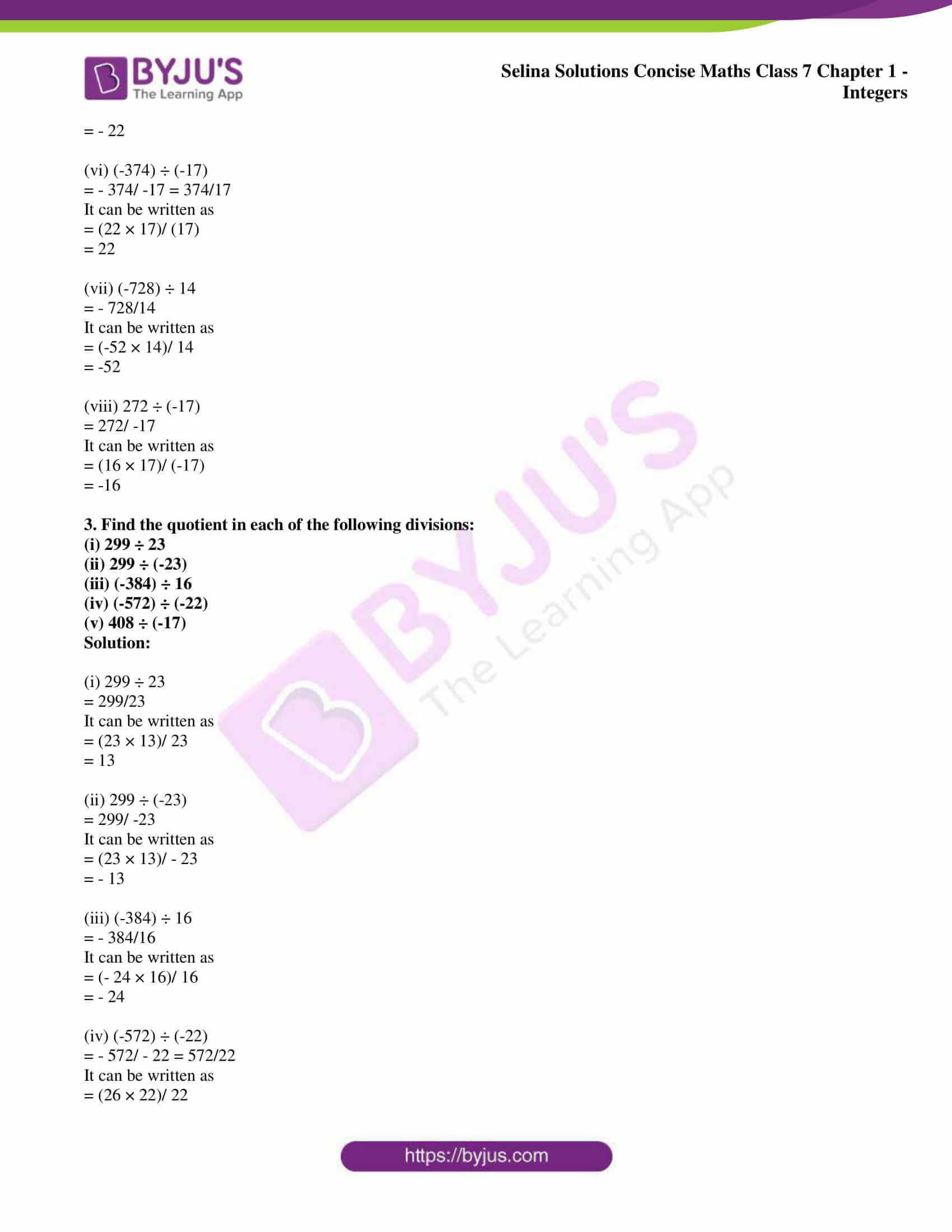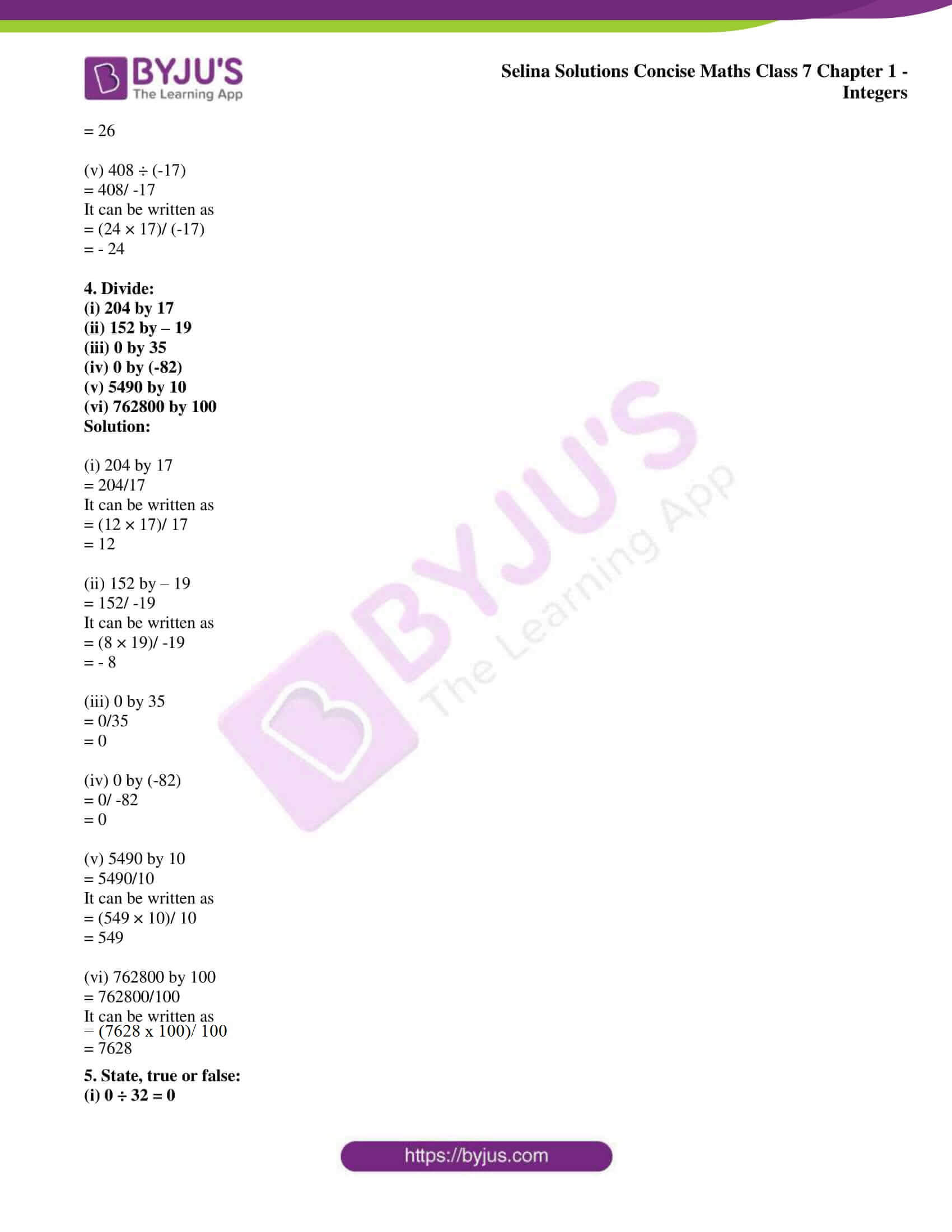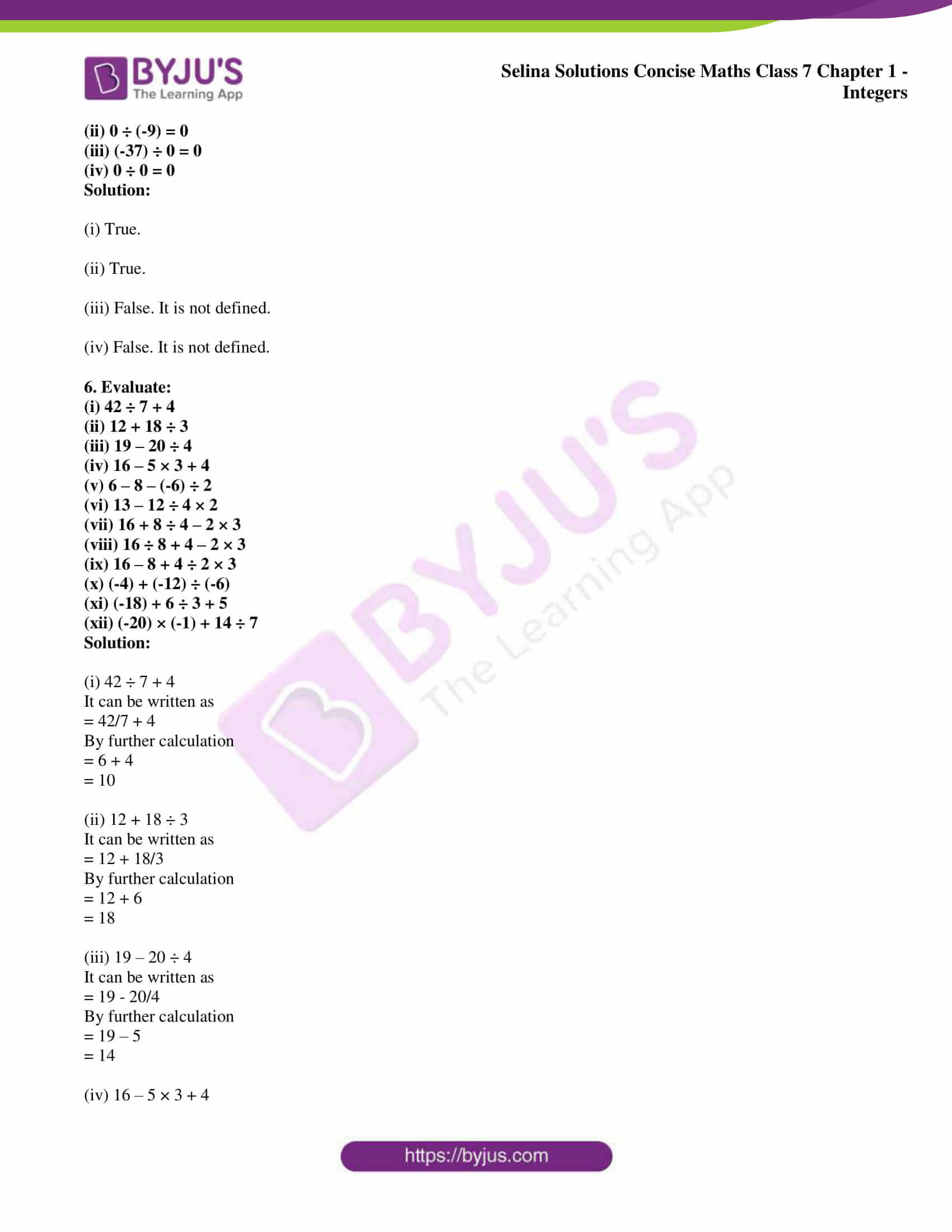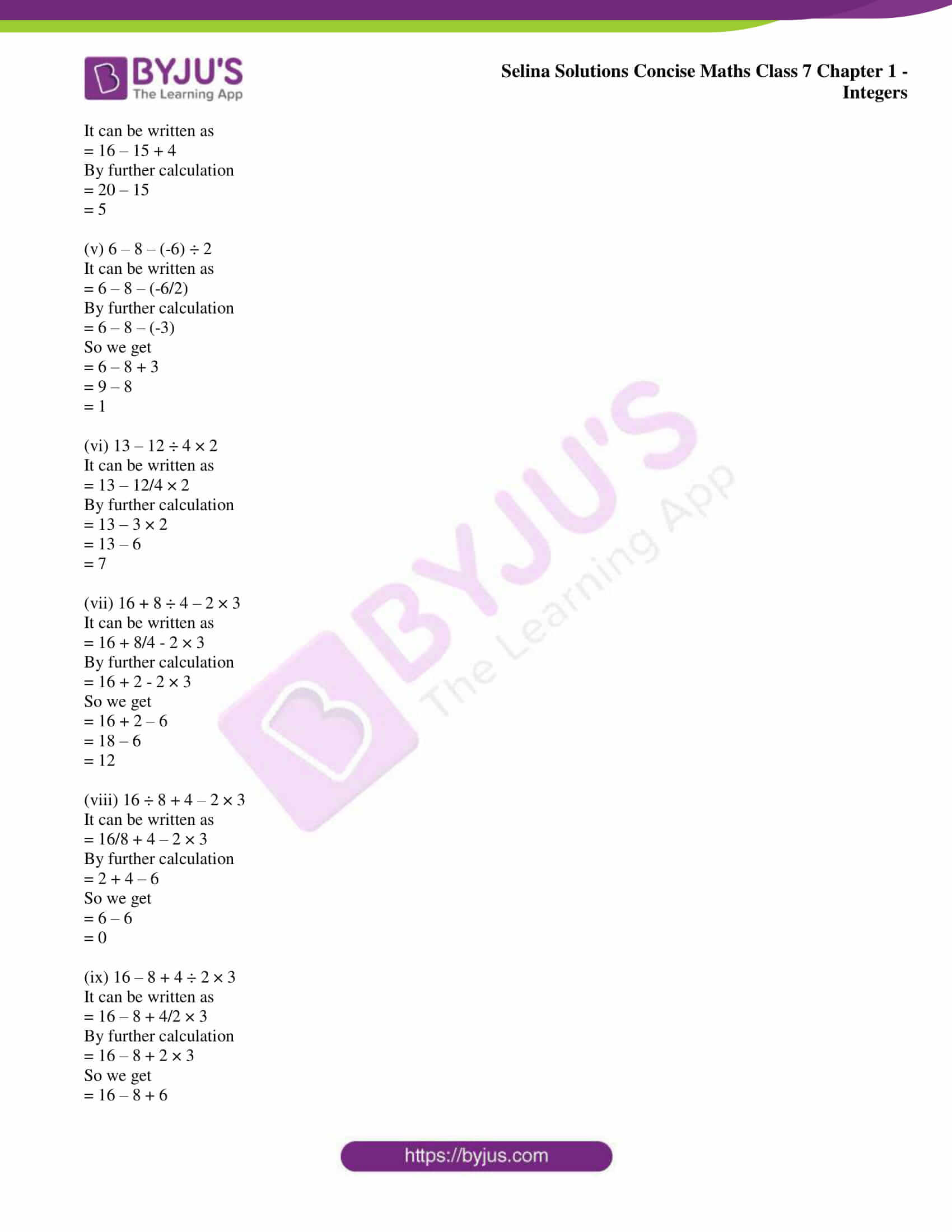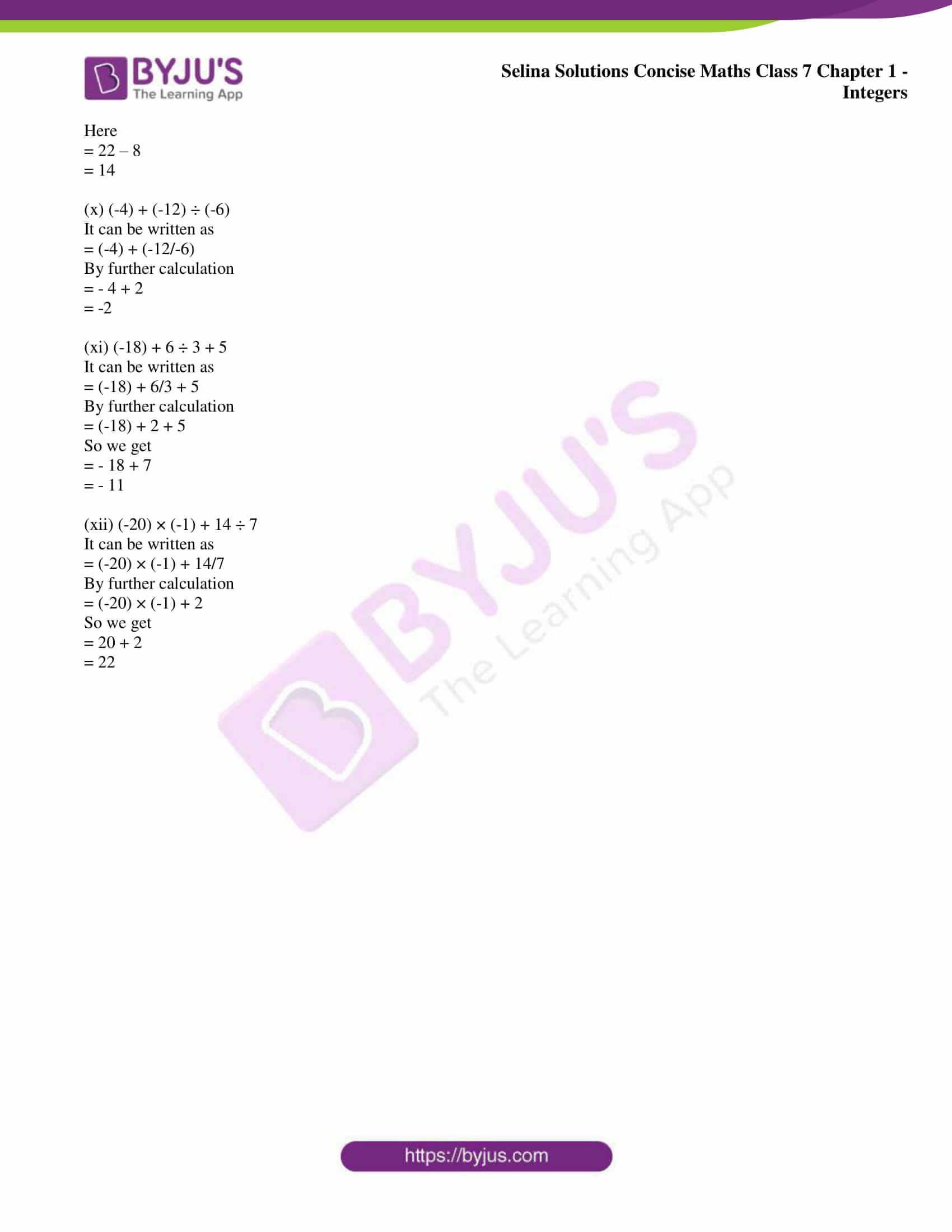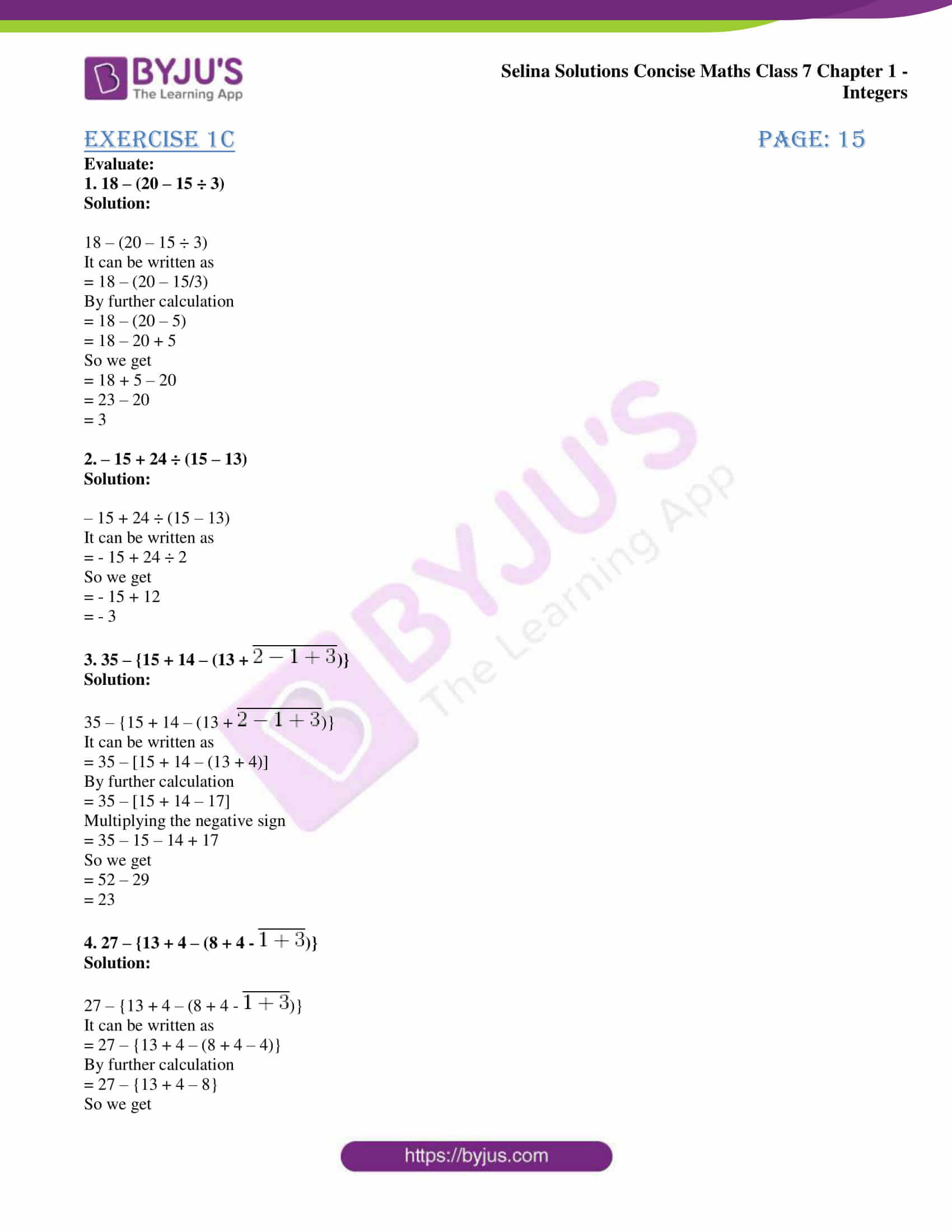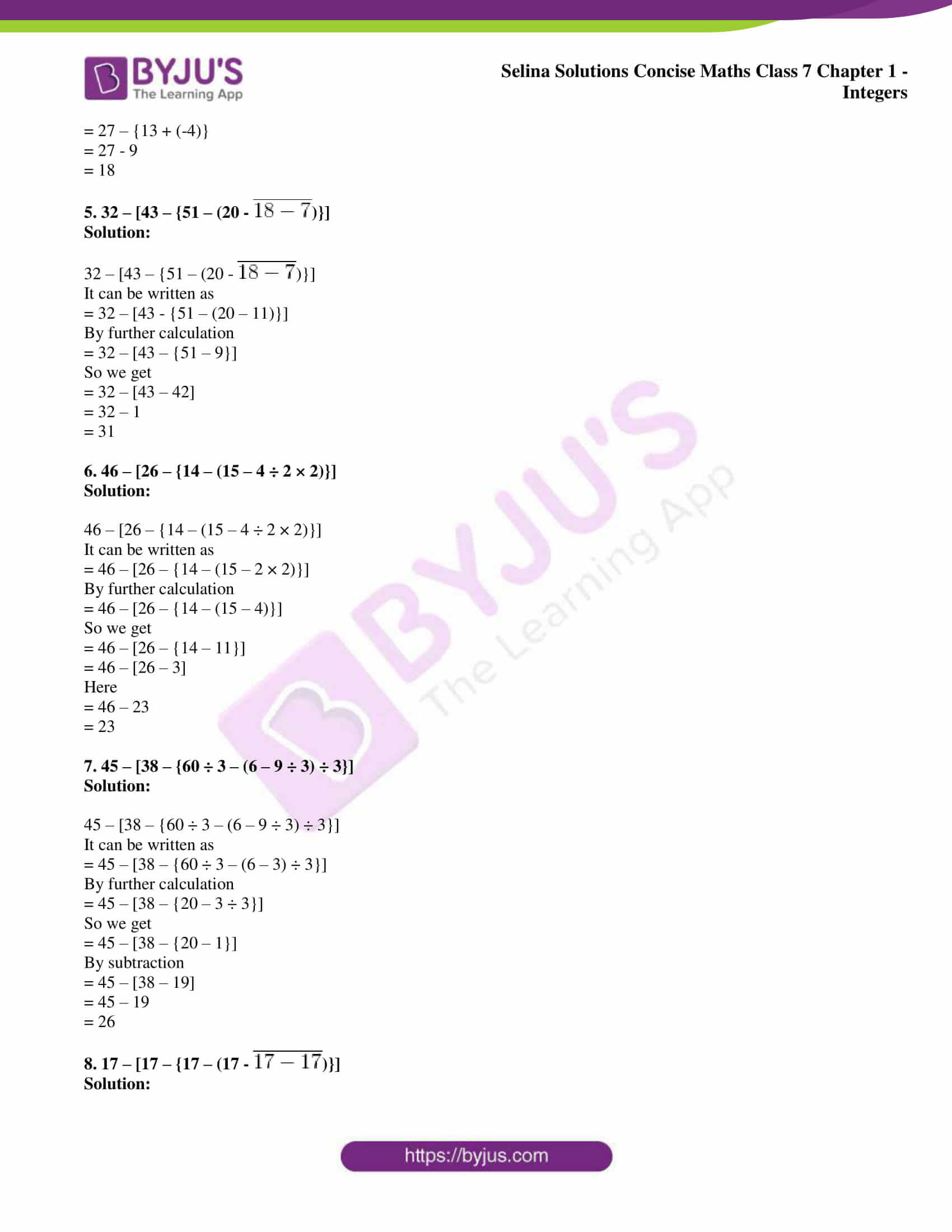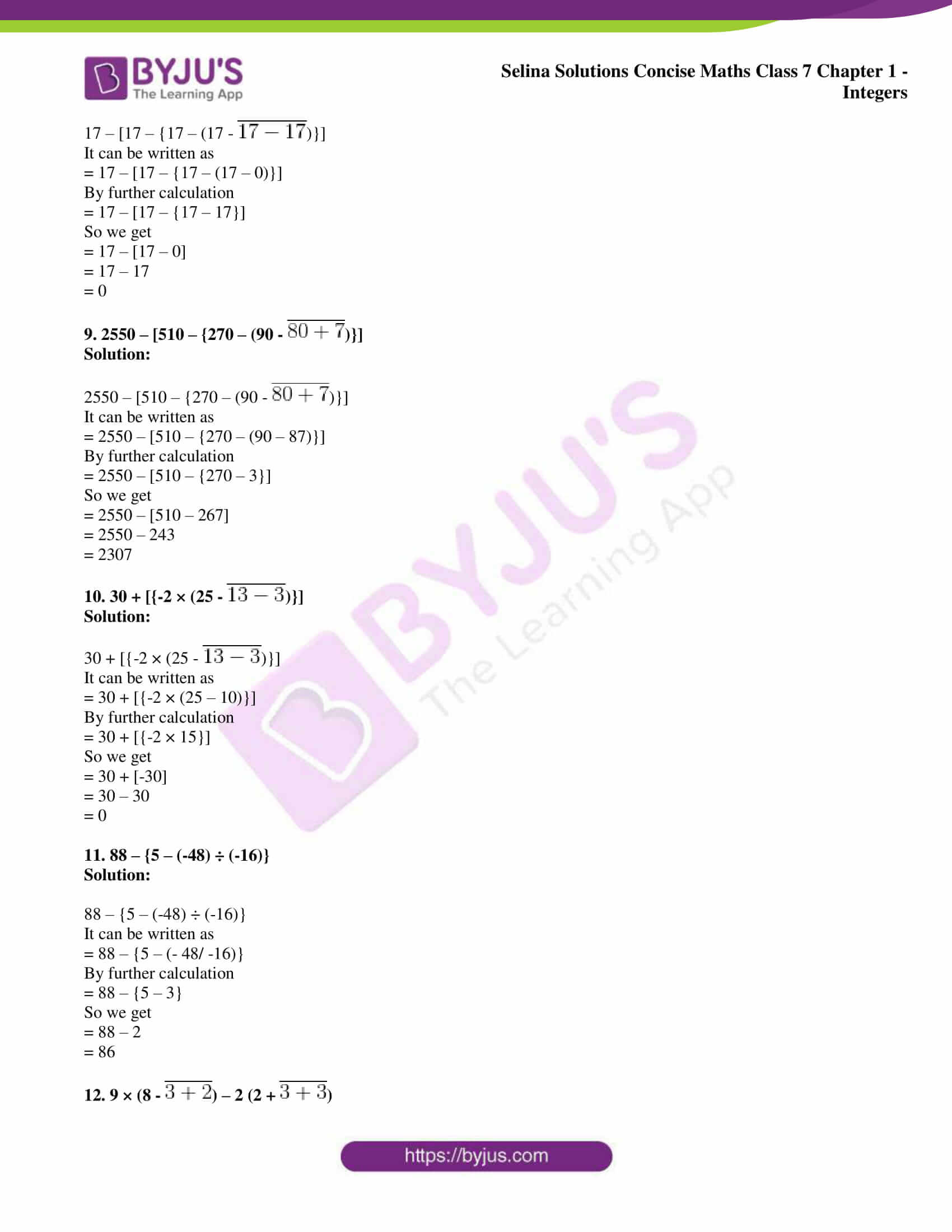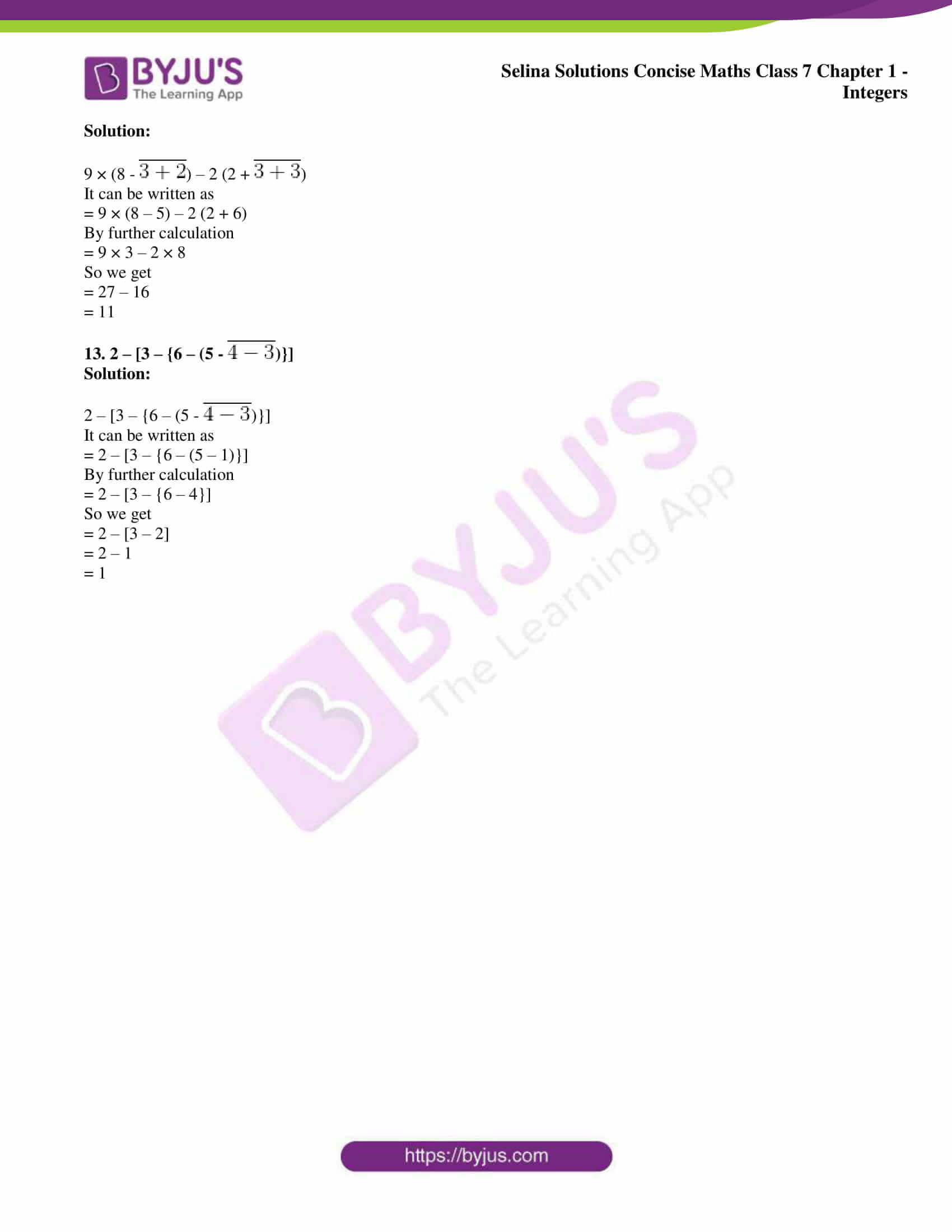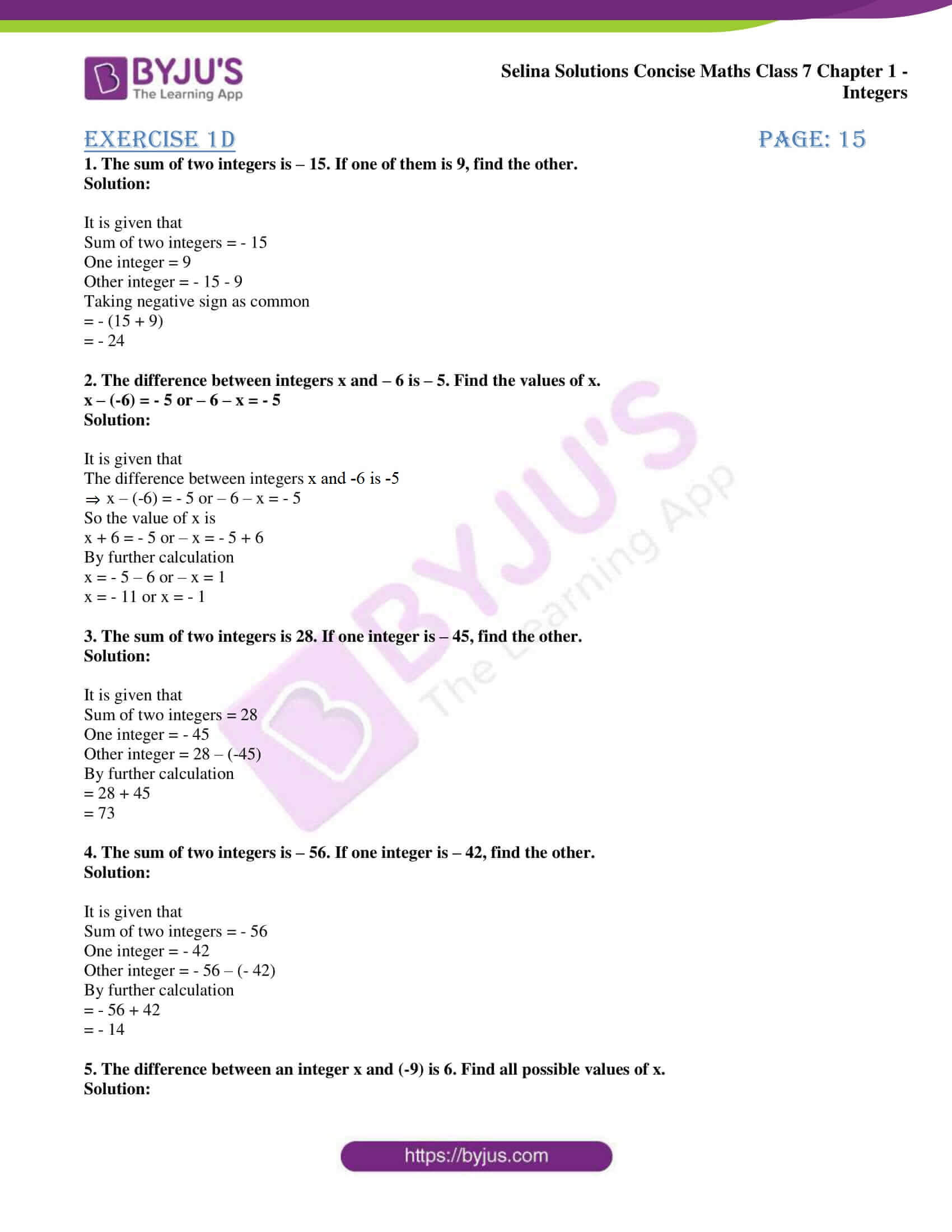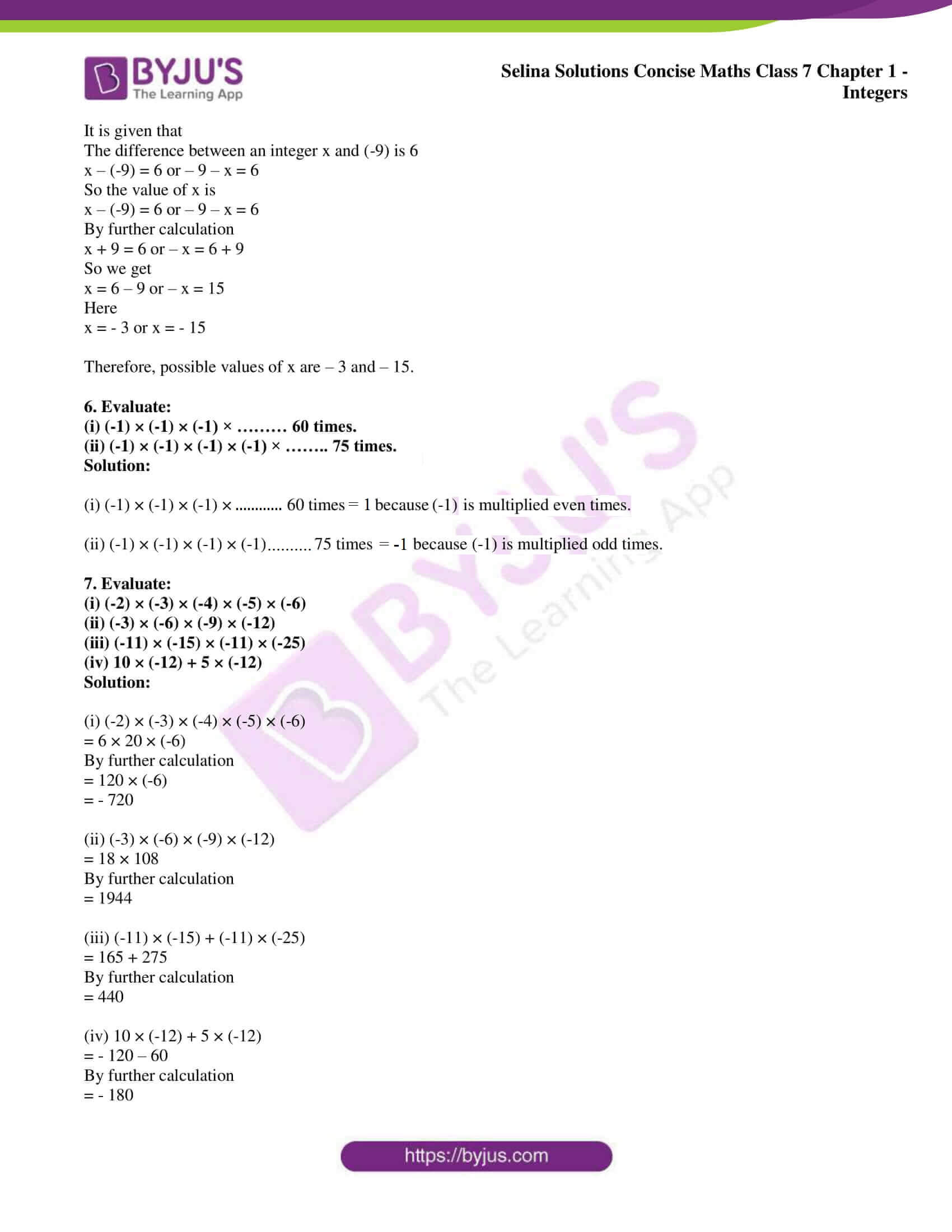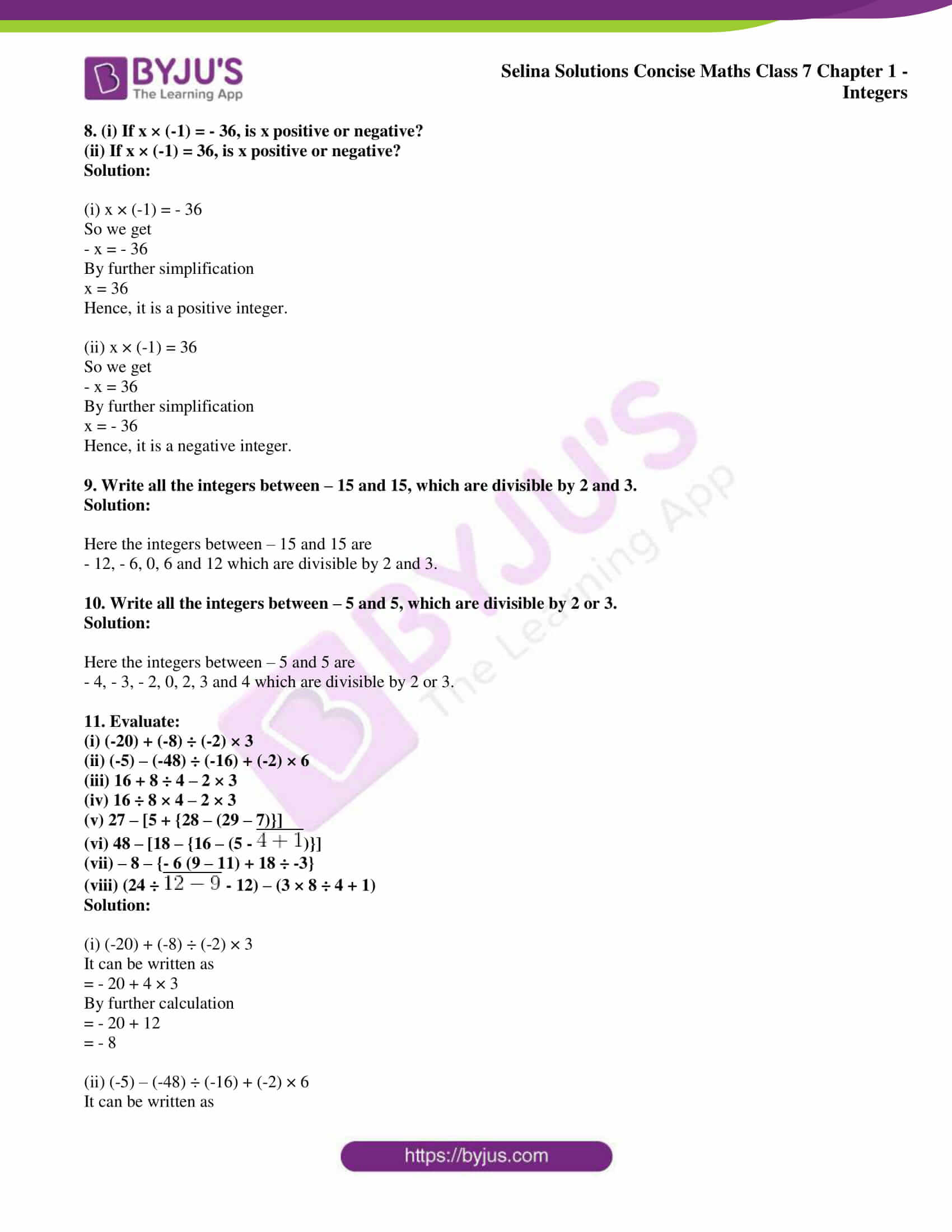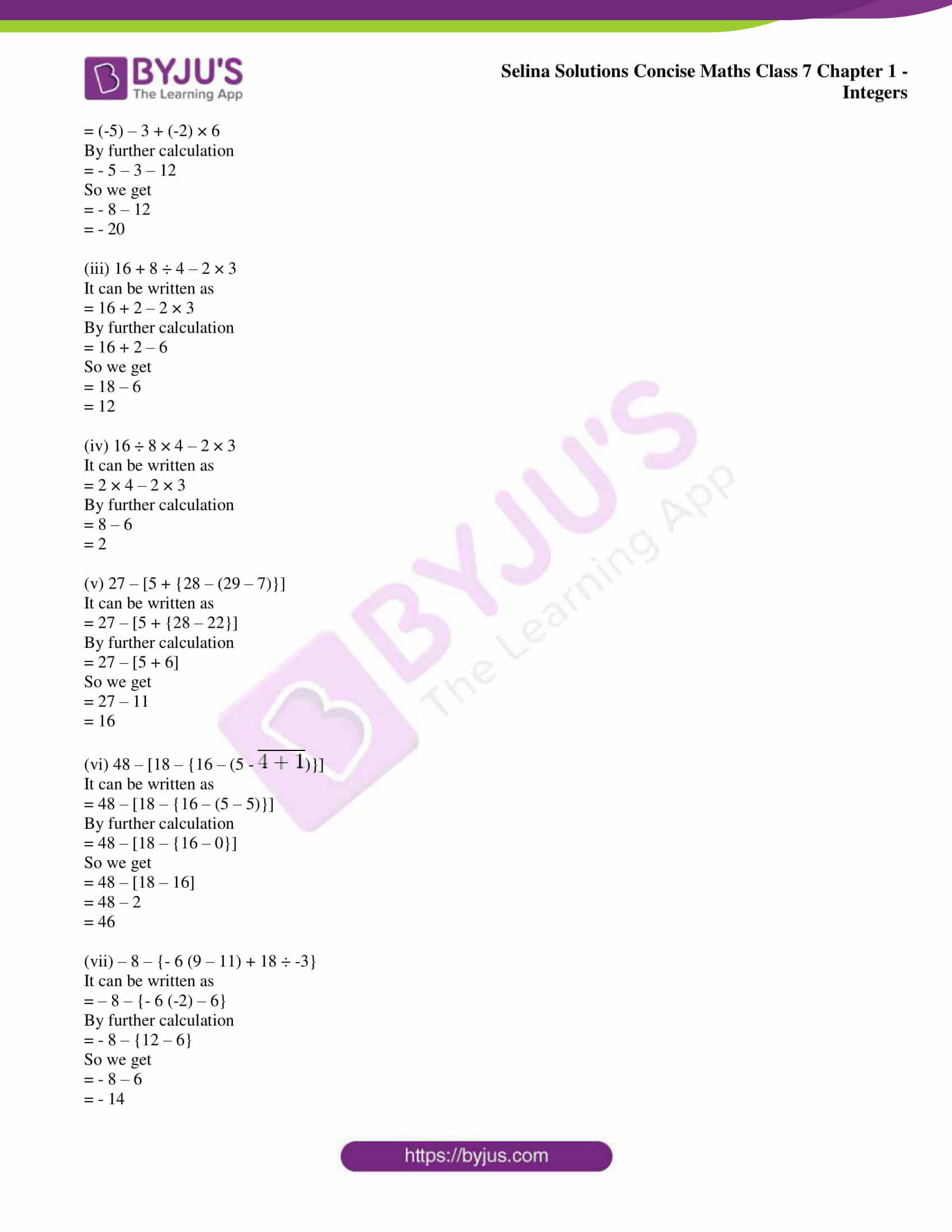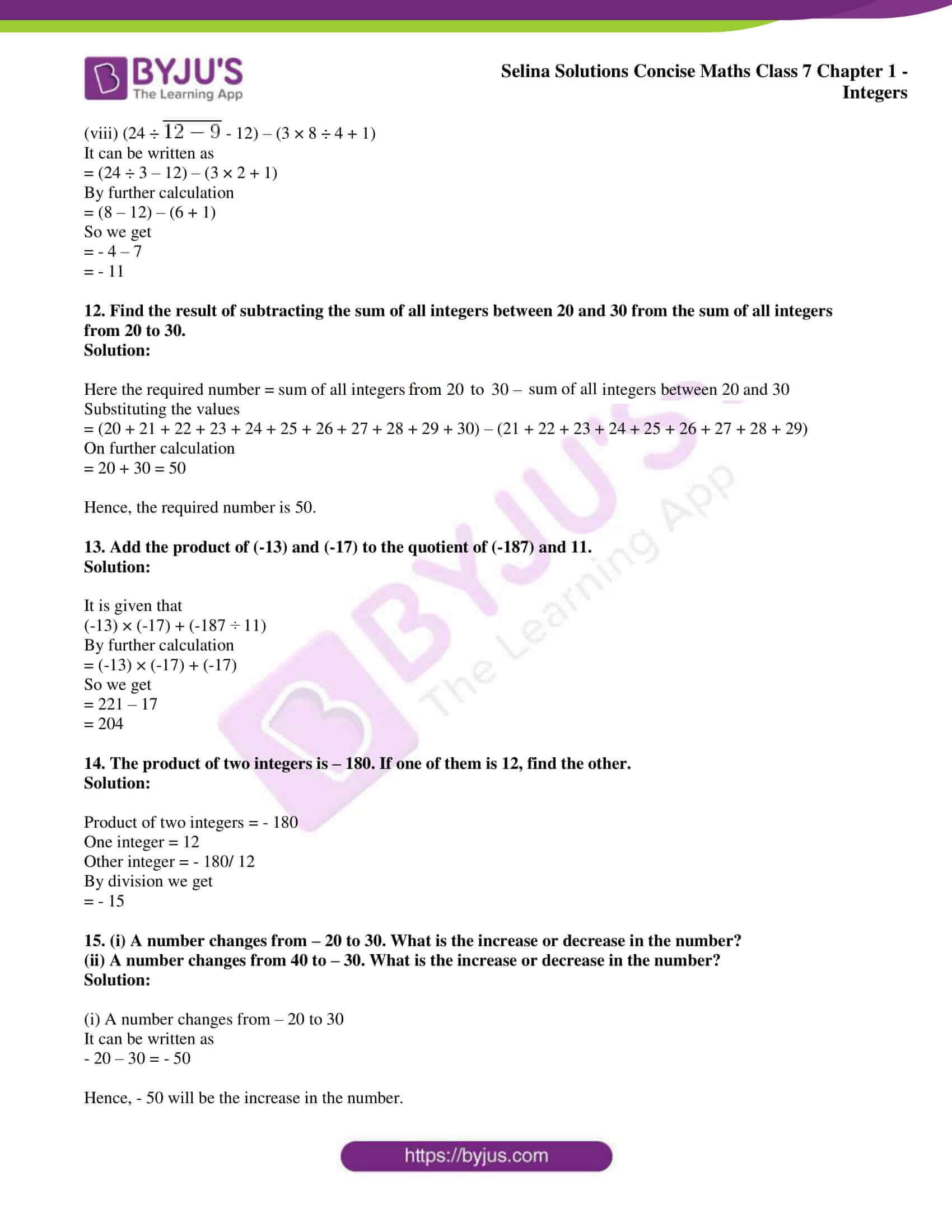### Exercises of Selina Solutions Concise Maths Class 7 Chapter 1 – Integers

Exercise 1A Solutions

Exercise 1B Solutions

Exercise 1C Solutions

Exercise 1D Solutions

## Access Selina Solutions Concise Maths Class 7 Chapter 1: Integers

#### Exercise 1A page: 6

1. Evaluate:

(i) 427 × 8 + 2 × 427

(ii) 394 × 12 + 394 × (-2)

(iii) 558 × 27 + 3 × 558

Solution:

(i) 427 × 8 + 2 × 427

Using Distributive property

= 427 × (8 + 2)

By further calculation

= 427 × 10

= 4270

(ii) 394 × 12 + 394 × (-2)

Using Distributive property

= 394 × (12 – 2)

By further calculation

= 394 × 10

= 3940

(iii) 558 × 27 + 3 × 558

Using Distributive property

= 558 × (27 + 3)

By further calculation

= 558 × 30

= 16740

2. Evaluate:

(i) 673 × 9 + 673

(ii) 1925 × 101 – 1925

Solution:

(i) 673 × 9 + 673

Using Distributive property

= 673 × (9 + 1)

By further calculation

= 673 × 10

= 6730

(ii) 1925 × 101 – 1925

Using Distributive property

= 1925 × (101 – 1)

By further calculation

= 1925 × 100

= 192500

3. Verify:

(i) 37 × {8 + (-3)} = 37 × 8 + 37 × (-3)

(ii) (-82) × {(- 4) + 19} = (-82) × (- 4) + (-82) × 19

(iii) {7 – (-7)} × 7 = 7 × 7 – (-7) × 7

(iv) {(-15) – 8} × -6 = (-15) × (-6) – 8 × (-6)

Solution:

(i) 37 × {8 + (-3)} = 37 × 8 + 37 × (-3)

Consider LHS = 37 × {8 + (-3)}

It can be written as

= 37 × {8 – 3}

By further calculation

= 37 × {5}

= 185

Similarly RHS = 37 × 8 + 37 × (-3)

It can be written as

= 37 × (8 – 3)

By further calculation

= 37 × 5

= 185

Therefore, LHS = RHS.

(ii) (-82) × {(- 4) + 19} = (-82) × (- 4) + (-82) × 19

Consider LHS = (-82) × {(- 4) + 19}

It can be written as

= (-82) × {-4 + 19}

By further calculation

= (-82) × {15}

= – 1230

Similarly RHS = (-82) × (- 4) + (-82) × 19

It can be written as

= (-82) × (-4 + 19)

By further calculation

= – 82 × 15

= – 1230

Therefore, LHS = RHS.

(iii) {7 – (-7)} × 7 = 7 × 7 – (-7) × 7

Consider LHS = {7 – (-7)} × 7

It can be written as

= {7 + 7} × 7

By further calculation

= {14} × 7

= 98

Similarly RHS = 7 × 7 – (-7) × 7

It can be written as

= 7 × 7 + 7 × 7

By further calculation

= 7 × (7 + 7)

= 7 × 14

= 98

Therefore, LHS = RHS.

(iv) {(-15) – 8} × -6 = (-15) × (-6) – 8 × (-6)

Consider LHS = {(-15) – 8} × -6

It can be written as

= {- 15 – 8} × -6

By further calculation

= {-23} × -6

= 138

Similarly RHS = (-15) × (-6) – 8 × (-6)

It can be written as

= – 6 × (- 15 – 8)

By further calculation

= – 6 × – 23

= 138

Therefore, LHS = RHS.

4. Evaluate:

(i) 15 × 8

(ii) 15 × (-8)

(iii) (-15) × 8

(iv) (-15) × -8

Solution:

(i) 15 × 8 = 120

(ii) 15 × (-8) = – 120

(iii) (-15) × 8 = – 120

(iv) (-15) × -8 = 120

5. Evaluate:

(i) 4 × 6 × 8

(ii) 4 × 6 × (-8)

(iii) 4 × (-6) × 8

(iv) (-4) × 6 × 8

(v) 4 × (-6) × (-8)

(vi) (-4) × (-6) × 8

(vii) (-4) × 6 × (-8)

(viii) (-4) × (-6) × (-8)

Solution:

(i) 4 × 6 × 8 = 192

(ii) 4 × 6 × (-8) = – 192

It has one negative factor

(iii) 4 × (-6) × 8 = – 192

It has one negative factor

(iv) (-4) × 6 × 8 = – 192

It has one negative factor

(v) 4 × (-6) × (-8) = 192

It has two negative factors

(vi) (-4) × (-6) × 8 = 192

It has two negative factors

(vii) (-4) × 6 × (-8) = 192

It has two negative factors

(viii) (-4) × (-6) × (-8) = – 192

It has three negative factors

6. Evaluate:

(i) 2 × 4 × 6 × 8

(ii) 2 × (-4) × 6 × 8

(iii) (-2) × 4 × (-6) × 8

(iv) (-2) × (-4) × 6 × (-8)

(v) (-2) × (-4) × (-6) × (-8)

Solution:

(i) 2 × 4 × 6 × 8 = 384

(ii) 2 × (-4) × 6 × 8 = -384

The number of negative integer in the product is odd

(iii) (-2) × 4 × (-6) × 8 = 384

The number of negative integer in the product is even

(iv) (-2) × (-4) × 6 × (-8) = -384

The number of negative integer in the product is odd

(v) (-2) × (-4) × (-6) × (-8) = 384

The number of negative integer in the product is even

7. Determine the integer whose product with ‘-1’ is:

(i) -47

(ii) 63

(iii) -1

(iv) 0

Solution:

(i) -47 = – 1 × 47

Therefore, the integer is 47.

(ii) 63 = – 1 × – 63

Therefore, the integer is – 63.

(iii) -1 = – 1 × 1

Therefore, the integer is 1.

(iv) 0 = -1 × 0

Therefore, the integer is 0.

8. Eighteen integers are multiplied together. What will be the sign of their product, if:

(i) 15 of them are negative and 3 are positive?

(ii) 12 of them are negative and 6 are positive?

(iii) 9 of them are positive and the remaining are negative?

(iv) all are negative?

Solution:

(i) Out of 18 integers, 15 of them are negative which is odd number. Therefore, the sign of product is negative.

(ii) Out of 18 integers, 12 of them are negative which is even number. Therefore, the sign of product is positive.

(iii) Out of 18 integers, 9 of them are negative which is odd number. Therefore, the sign of product is negative.

(iv) All are negative which is even number. Therefore, sign of product is positive.

9. Find which is greater?

(i) (8 + 10) × 15 or 8 + 10 × 15

(ii) 12 × (6 – 8) or 12 × 6 – 8

(iii) {(-3) – 4} × (-5) or (-3) – 4 × (-5)

Solution:

(i) (8 + 10) × 15 or 8 + 10 × 15

We know that

(8 + 10) × 15 = 18 × 15 = 270

8 + 10 × 15 = 8 + 150 = 158

Therefore, (8 + 10) × 15 > 8 + 10 × 15.

(ii) 12 × (6 – 8) or 12 × 6 – 8

We know that

12 × (6 – 8) = 12 (-2) = – 24

12 × 6 – 8 = 72 – 8 = 64

Therefore, 12 × (6 – 8) < 12 × 6 – 8.

(iii) {(-3) – 4} × (-5) or (-3) – 4 × (-5)

We know that

{(-3) – 4} × (-5) = {- 3 – 4} × (-5)

By further calculation

= – 7 × -5 = 35

Similarly

(-3) – 4 × (-5) = – 3 + 20 = 17

Therefore, {(-3) – 4} × (-5) > (-3) – 4 × (-5)

10. State, true or false:

(i) product of two different integers can be zero.

(ii) product of 120 negative integers and 121 positive integers is negative.

(iii) a × (b + c) = a × b + c

(iv) (b – c) × a = b – c × a.

Solution:

(i) True.

Example: 5 × 0 = 0, 0 × – 8 = 0

(ii) False.

The total number of negative integers is 120 which is an even number and we know that the product of even numbers of negative integers is always positive. Hence, the sign of the product will be positive.

(iii) False.

a × (b + c) ≠ a × b + c

ab + ac ≠ ab + c

(iv) False.

(b – c) × a ≠ b – c × a

ab – ac ≠ b – ca

#### Exercise 1B page: 11

1. Divide:

(i) 117 by 9

(ii) (-117) by 9

(iii) 117 by (-9)

(iv) (-117) by (-9)

(v) 225 by (-15)

(vi) (-552) ÷ 24

(vii) (-798) by (-21)

(viii) (-910) ÷ 26

Solution:

(i) 117 by 9

= 117/9

It can be written as

= (13 × 9)/ 9

= 13

(ii) (-117) by 9

= – 117/ 9

It can be written as

= (-13 × 9)/ 9

= -13

(iii) 117 by (-9)

= 117/ -9

It can be written as

= (13 × 9)/ -9

= – 13

(iv) (-117) by (-9)

= – 117/-9 = 117/9

It can be written as

= (13 × 9)/ 9

= 13

(v) 225 by (-15)

= 225/ (-15)

It can be written as

= (15 × 15)/ -15

= – 15

(vi) (-552) ÷ 24

= – 552/ 24

It can be written as

= (- 23 × 24)/ 24

= – 23

(vii) (-798) by (-21)

= – 798/ – 21 = 798/21

It can be written as

= (38 × 21)/ 21

= 38

(viii) (-910) ÷ 26

= – 910/ 26

It can be written as

= (- 35 × 26)/ 26

= – 35

2. Evaluate:

(i) (-234) ÷ 13

(ii) 234 ÷ (-13)

(iii) (-234) ÷ (-13)

(iv) 374 ÷ (-17)

(v) (-374) ÷ 17

(vi) (-374) ÷ (-17)

(vii) (-728) ÷ 14

(viii) 272 ÷ (-17)

Solution:

(i) (-234) ÷ 13

= – 234/ 13

It can be written as

= (-18 × 13)/ 13

= – 18

(ii) 234 ÷ (-13)

= 234/ -13

It can be written as

= (18 × 13)/ – 13

= – 18

(iii) (-234) ÷ (-13)

= – 234/ – 13 = 234/13

It can be written as

= (18 × 13)/ 13

= 18

(iv) 374 ÷ (-17)

= 374/ -17

It can be written as

= (22 × 17)/ (-17)

= – 22

(v) (-374) ÷ 17

= – 374/ 17

It can be written as

= (-22 × 17)/ (17)

= – 22

(vi) (-374) ÷ (-17)

= – 374/ -17 = 374/17

It can be written as

= (22 × 17)/ (17)

= 22

(vii) (-728) ÷ 14

= – 728/14

It can be written as

= (-52 × 14)/ 14

= -52

(viii) 272 ÷ (-17)

= 272/ -17

It can be written as

= (16 × 17)/ (-17)

= -16

3. Find the quotient in each of the following divisions:

(i) 299 ÷ 23

(ii) 299 ÷ (-23)

(iii) (-384) ÷ 16

(iv) (-572) ÷ (-22)

(v) 408 ÷ (-17)

Solution:

(i) 299 ÷ 23

= 299/23

It can be written as

= (23 × 13)/ 23

= 13

(ii) 299 ÷ (-23)

= 299/ -23

It can be written as

= (23 × 13)/ – 23

= – 13

(iii) (-384) ÷ 16

= – 384/16

It can be written as

= (- 24 × 16)/ 16

= – 24

(iv) (-572) ÷ (-22)

= – 572/ – 22 = 572/22

It can be written as

= (26 × 22)/ 22

= 26

(v) 408 ÷ (-17)

= 408/ -17

It can be written as

= (24 × 17)/ (-17)

= – 24

4. Divide:

(i) 204 by 17

(ii) 152 by – 19

(iii) 0 by 35

(iv) 0 by (-82)

(v) 5490 by 10

(vi) 762800 by 100

Solution:

(i) 204 by 17

= 204/17

It can be written as

= (12 × 17)/ 17

= 12

(ii) 152 by – 19

= 152/ -19

It can be written as

= (8 × 19)/ -19

= – 8

(iii) 0 by 35

= 0/35

= 0

(iv) 0 by (-82)

= 0/ -82

= 0

(v) 5490 by 10

= 5490/10

It can be written as

= (549 × 10)/ 10

= 549

(vi) 762800 by 100

= 762800/100

It can be written as

= (7628 x 100)/ 100

= 7628

5. State, true or false:

(i) 0 ÷ 32 = 0

(ii) 0 ÷ (-9) = 0

(iii) (-37) ÷ 0 = 0

(iv) 0 ÷ 0 = 0

Solution:

(i) True.

(ii) True.

(iii) False. It is not defined.

(iv) False. It is not defined.

6. Evaluate:

(i) 42 ÷ 7 + 4

(ii) 12 + 18 ÷ 3

(iii) 19 – 20 ÷ 4

(iv) 16 – 5 × 3 + 4

(v) 6 – 8 – (-6) ÷ 2

(vi) 13 – 12 ÷ 4 × 2

(vii) 16 + 8 ÷ 4 – 2 × 3

(viii) 16 ÷ 8 + 4 – 2 × 3

(ix) 16 – 8 + 4 ÷ 2 × 3

(x) (-4) + (-12) ÷ (-6)

(xi) (-18) + 6 ÷ 3 + 5

(xii) (-20) × (-1) + 14 ÷ 7

Solution:

(i) 42 ÷ 7 + 4

It can be written as

= 42/7 + 4

By further calculation

= 6 + 4

= 10

(ii) 12 + 18 ÷ 3

It can be written as

= 12 + 18/3

By further calculation

= 12 + 6

= 18

(iii) 19 – 20 ÷ 4

It can be written as

= 19 – 20/4

By further calculation

= 19 – 5

= 14

(iv) 16 – 5 × 3 + 4

It can be written as

= 16 – 15 + 4

By further calculation

= 20 – 15

= 5

(v) 6 – 8 – (-6) ÷ 2

It can be written as

= 6 – 8 – (-6/2)

By further calculation

= 6 – 8 – (-3)

So we get

= 6 – 8 + 3

= 9 – 8

= 1

(vi) 13 – 12 ÷ 4 × 2

It can be written as

= 13 – 12/4 × 2

By further calculation

= 13 – 3 × 2

= 13 – 6

= 7

(vii) 16 + 8 ÷ 4 – 2 × 3

It can be written as

= 16 + 8/4 – 2 × 3

By further calculation

= 16 + 2 – 2 × 3

So we get

= 16 + 2 – 6

= 18 – 6

= 12

(viii) 16 ÷ 8 + 4 – 2 × 3

It can be written as

= 16/8 + 4 – 2 × 3

By further calculation

= 2 + 4 – 6

So we get

= 6 – 6

= 0

(ix) 16 – 8 + 4 ÷ 2 × 3

It can be written as

= 16 – 8 + 4/2 × 3

By further calculation

= 16 – 8 + 2 × 3

So we get

= 16 – 8 + 6

Here

= 22 – 8

= 14

(x) (-4) + (-12) ÷ (-6)

It can be written as

= (-4) + (-12/-6)

By further calculation

= – 4 + 2

= -2

(xi) (-18) + 6 ÷ 3 + 5

It can be written as

= (-18) + 6/3 + 5

By further calculation

= (-18) + 2 + 5

So we get

= – 18 + 7

= – 11

(xii) (-20) × (-1) + 14 ÷ 7

It can be written as

= (-20) × (-1) + 14/7

By further calculation

= (-20) × (-1) + 2

So we get

= 20 + 2

= 22

#### Exercise 1C page: 15

Evaluate:

1. 18 – (20 – 15 ÷ 3)

Solution:

18 – (20 – 15 ÷ 3)

It can be written as

= 18 – (20 – 15/3)

By further calculation

= 18 – (20 – 5)

= 18 – 20 + 5

So we get

= 18 + 5 – 20

= 23 – 20

= 3

2. – 15 + 24 ÷ (15 – 13)

Solution:

– 15 + 24 ÷ (15 – 13)

It can be written as

= – 15 + 24 ÷ 2

So we get

= – 15 + 12

= – 3

3. 35 – {15 + 14 – (13 +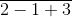)}

Solution:

35 – {15 + 14 – (13 +)}

It can be written as

= 35 – [15 + 14 – (13 + 4)]

By further calculation

= 35 – [15 + 14 – 17]

Multiplying the negative sign

= 35 – 15 – 14 + 17

So we get

= 52 – 29

= 23

4. 27 – {13 + 4 – (8 + 4 –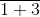)}

Solution:

27 – {13 + 4 – (8 + 4 –)}

It can be written as

= 27 – {13 + 4 – (8 + 4 – 4)}

By further calculation

= 27 – {13 + 4 – 8}

So we get

= 27 – {13 + (-4)}

= 27 – 9

= 18

5. 32 – [43 – {51 – (20 –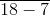)}]

Solution:

32 – [43 – {51 – (20 –)}]

It can be written as

= 32 – [43 – {51 – (20 – 11)}]

By further calculation

= 32 – [43 – {51 – 9}]

So we get

= 32 – [43 – 42]

= 32 – 1

= 31

6. 46 – [26 – {14 – (15 – 4 ÷ 2 × 2)}]

Solution:

46 – [26 – {14 – (15 – 4 ÷ 2 × 2)}]

It can be written as

= 46 – [26 – {14 – (15 – 2 × 2)}]

By further calculation

= 46 – [26 – {14 – (15 – 4)}]

So we get

= 46 – [26 – {14 – 11}]

= 46 – [26 – 3]

Here

= 46 – 23

= 23

7. 45 – [38 – {60 ÷ 3 – (6 – 9 ÷ 3) ÷ 3}]

Solution:

45 – [38 – {60 ÷ 3 – (6 – 9 ÷ 3) ÷ 3}]

It can be written as

= 45 – [38 – {60 ÷ 3 – (6 – 3) ÷ 3}]

By further calculation

= 45 – [38 – {20 – 3 ÷ 3}]

So we get

= 45 – [38 – {20 – 1}]

By subtraction

= 45 – [38 – 19]

= 45 – 19

= 26

8. 17 – [17 – {17 – (17 –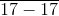)}]

Solution:

17 – [17 – {17 – (17 –)}]

It can be written as

= 17 – [17 – {17 – (17 – 0)}]

By further calculation

= 17 – [17 – {17 – 17}]

So we get

= 17 – [17 – 0]

= 17 – 17

= 0

9. 2550 – [510 – {270 – (90 –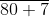)}]

Solution:

2550 – [510 – {270 – (90 –)}]

It can be written as

= 2550 – [510 – {270 – (90 – 87)}]

By further calculation

= 2550 – [510 – {270 – 3}]

So we get

= 2550 – [510 – 267]

= 2550 – 243

= 2307

10. 30 + [{-2 × (25 –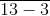)}]

Solution:

30 + [{-2 × (25 –)}]

It can be written as

= 30 + [{-2 × (25 – 10)}]

By further calculation

= 30 + [{-2 × 15}]

So we get

= 30 + [-30]

= 30 – 30

= 0

11. 88 – {5 – (-48) ÷ (-16)}

Solution:

88 – {5 – (-48) ÷ (-16)}

It can be written as

= 88 – {5 – (- 48/ -16)}

By further calculation

= 88 – {5 – 3}

So we get

= 88 – 2

= 86

12. 9 × (8 –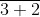) – 2 (2 +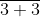)

Solution:

9 × (8 –) – 2 (2 +)

It can be written as

= 9 × (8 – 5) – 2 (2 + 6)

By further calculation

= 9 × 3 – 2 × 8

So we get

= 27 – 16

= 11

13. 2 – [3 – {6 – (5 –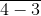)}]

Solution:

2 – [3 – {6 – (5 –)}]

It can be written as

= 2 – [3 – {6 – (5 – 1)}]

By further calculation

= 2 – [3 – {6 – 4}]

So we get

= 2 – [3 – 2]

= 2 – 1

= 1

#### Exercise 1D page: 15

1. The sum of two integers is – 15. If one of them is 9, find the other.

Solution:

It is given that

Sum of two integers = – 15

One integer = 9

Other integer = – 15 – 9

Taking negative sign as common

= – (15 + 9)

= – 24

2. The difference between integers x and – 6 is – 5. Find the values of x.

x – (-6) = – 5 or – 6 – x = – 5

Solution:

It is given that

The difference between integers x and -6 is -5

x – (-6) = – 5 or – 6 – x = – 5

So the value of x is

x + 6 = – 5 or – x = – 5 + 6

By further calculation

x = – 5 – 6 or – x = 1

x = – 11 or x = – 1

3. The sum of two integers is 28. If one integer is – 45, find the other.

Solution:

It is given that

Sum of two integers = 28

One integer = – 45

Other integer = 28 – (-45)

By further calculation

= 28 + 45

= 73

4. The sum of two integers is – 56. If one integer is – 42, find the other.

Solution:

It is given that

Sum of two integers = – 56

One integer = – 42

Other integer = – 56 – (- 42)

By further calculation

= – 56 + 42

= – 14

5. The difference between an integer x and (-9) is 6. Find all possible values of x.

Solution:

It is given that

The difference between an integer x and (-9) is 6

x – (-9) = 6 or – 9 – x = 6

So the value of x is

x – (-9) = 6 or – 9 – x = 6

By further calculation

x + 9 = 6 or – x = 6 + 9

So we get

x = 6 – 9 or – x = 15

Here

x = – 3 or x = – 15

Therefore, possible values of x are – 3 and – 15.

6. Evaluate:

(i) (-1) × (-1) × (-1) × ……… 60 times.

(ii) (-1) × (-1) × (-1) × (-1) × …….. 75 times.

Solution:

(i) (-1) × (-1) × (-1) × ……… 60 times = 1 because (-1) is multiplied even number of times.

(ii) (-1) × (-1) × (-1) × (-1) × ……… 75 times = -1 because (-1) is multiplied odd number of times.

7. Evaluate:

(i) (-2) × (-3) × (-4) × (-5) × (-6)

(ii) (-3) × (-6) × (-9) × (-12)

(iii) (-11) × (-15) × (-11) × (-25)

(iv) 10 × (-12) + 5 × (-12)

Solution:

(i) (-2) × (-3) × (-4) × (-5) × (-6)

= 6 × 20 × (-6)

By further calculation

= 120 × (-6)

= – 720

(ii) (-3) × (-6) × (-9) × (-12)

= 18 × 108

By further calculation

= 1944

(iii) (-11) × (-15) + (-11) × (-25)

= 165 + 275

By further calculation

= 440

(iv) 10 × (-12) + 5 × (-12)

= – 120 – 60

By further calculation

= – 180

8. (i) If x × (-1) = – 36, is x positive or negative?

(ii) If x × (-1) = 36, is x positive or negative?

Solution:

(i) x × (-1) = – 36

So we get

– x = – 36

By further simplification

x = 36

Hence, it is a positive integer.

(ii) x × (-1) = 36

So we get

– x = 36

By further simplification

x = – 36

Hence, it is a negative integer.

9. Write all the integers between – 15 and 15, which are divisible by 2 and 3.

Solution:

Here the integers between – 15 and 15 are

– 12, – 6, 0, 6 and 12 which are divisible by 2 and 3.

10. Write all the integers between – 5 and 5, which are divisible by 2 or 3.

Solution:

Here the integers between – 5 and 5 are

– 4, – 3, – 2, 0, 2, 3 and 4 which are divisible by 2 or 3.

11. Evaluate:

(i) (-20) + (-8) ÷ (-2) × 3

(ii) (-5) – (-48) ÷ (-16) + (-2) × 6

(iii) 16 + 8 ÷ 4 – 2 × 3

(iv) 16 ÷ 8 × 4 – 2 × 3

(v) 27 – [5 + {28 – (29 – 7)}]

(vi) 48 – [18 – {16 – (5 –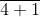)}]

(vii) – 8 – {- 6 (9 – 11) + 18 ÷ -3}

(viii) (24 ÷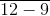– 12) – (3 × 8 ÷ 4 + 1)

Solution:

(i) (-20) + (-8) ÷ (-2) × 3

It can be written as

= – 20 + 4 × 3

By further calculation

= – 20 + 12

= – 8

(ii) (-5) – (-48) ÷ (-16) + (-2) × 6

It can be written as

= (-5) – 3 + (-2) × 6

By further calculation

= – 5 – 3 – 12

So we get

= – 8 – 12

= – 20

(iii) 16 + 8 ÷ 4 – 2 × 3

It can be written as

= 16 + 2 – 2 × 3

By further calculation

= 16 + 2 – 6

So we get

= 18 – 6

= 12

(iv) 16 ÷ 8 × 4 – 2 × 3

It can be written as

= 2 × 4 – 2 × 3

By further calculation

= 8 – 6

= 2

(v) 27 – [5 + {28 – (29 – 7)}]

It can be written as

= 27 – [5 + {28 – 22}]

By further calculation

= 27 – [5 + 6]

So we get

= 27 – 11

= 16

(vi) 48 – [18 – {16 – (5 –)}]

It can be written as

= 48 – [18 – {16 – (5 – 5)}]

By further calculation

= 48 – [18 – {16 – 0}]

So we get

= 48 – [18 – 16]

= 48 – 2

= 46

(vii) – 8 – {- 6 (9 – 11) + 18 ÷ -3}

It can be written as

= – 8 – {- 6 (-2) – 6}

By further calculation

= – 8 – {12 – 6}

So we get

= – 8 – 6

= – 14

(viii) (24 ÷– 12) – (3 × 8 ÷ 4 + 1)

It can be written as

= (24 ÷ 3 – 12) – (3 × 2 + 1)

By further calculation

= (8 – 12) – (6 + 1)

So we get

= – 4 – 7

= – 11

12. Find the result of subtracting the sum of all integers between 20 and 30 from the sum of all integers from 20 to 30.

Solution:

Here the required number = sum of all integers from 20 to 30 – sum of all integers between 20 and 30

Substituting the values

= (20 + 21 + 22 + 23 + 24 + 25 + 26 + 27 + 28 + 29 + 30) – (21 + 22 + 23 + 24 + 25 + 26 + 27 + 28 + 29)

On further calculation

= 20 + 30 = 50

Hence, the required number is 50.

13. Add the product of (-13) and (-17) to the quotient of (-187) and 11.

Solution:

It is given that

(-13) × (-17) + (-187 ÷ 11)

By further calculation

= (-13) × (-17) + (-17)

So we get

= 221 – 17

= 204

14. The product of two integers is – 180. If one of them is 12, find the other.

Solution:

Product of two integers = – 180

One integer = 12

Other integer = – 180/ 12

By division we get

= – 15

15. (i) A number changes from – 20 to 30. What is the increase or decrease in the number?

(ii) A number changes from 40 to – 30. What is the increase or decrease in the number?

Solution:

(i) A number changes from – 20 to 30

It can be written as

– 20 – 30 = – 50

Hence, – 50 will be the increase in the number.

(ii) A number changes from 40 to – 30

It can be written as

40 – (-30) = 40 + 30 = 70

Hence, 70 will be the decrease in the number.